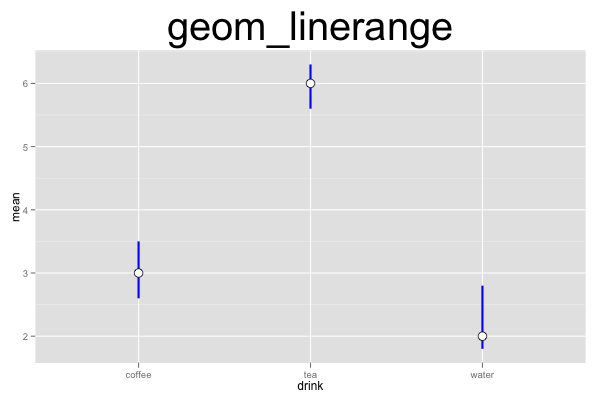## ggplot2 Quick Reference: geom_linerange

A geom that draws line ranges, defined by an upper and lower value. This is useful e.g., to draw confidence intervals.

A line range is similar to a pointrange (minus the point). It is also similar to an errorbar (minus the whiskers).

Default statistic: stat_identity

### Parameters

• x - (required) x coordinate of the line
• ymin - (required) y coordinate of the lower end of the line
• ymax - (required) y coordinate of the upper end of the line
• size - (default: 0.5) thickness of the lines
• linetype - (default: 1=solid) the type of the lines
• colour - (default: "black") the color of the lines
• width - (default: 0.9) width of the whiskers
• alpha - (default: 1=opaque) the transparency of the lines

### Example

This plot consists of two layers. The bottom layer shows line ranges, and the top layer shows points. Note that we have to provide (or compute) the ymin and ymax values for the line ranges ourselves (the linerange geom does not automatically compute e.g., a confidence interval).```d=data.frame(drink=c("coffee","tea","water"), mean=c(3,6,2), lower=c(2.6,5.6,1.8), upper=c(3.5,6.3,2.8))
ggplot() +
geom_linerange(data=d, mapping=aes(x=drink, ymin=upper, ymax=lower), width=0.2, size=1, color="blue") +
geom_point(data=d, mapping=aes(x=drink, y=mean), size=4, shape=21, fill="white") +
opts(title="geom_linerange", plot.title=theme_text(size=40, vjust=1.5))```